# Linear Functions

Parent topic:
Functions
•### Graph the Line

Activity

Steve Phelps

•### Over 240 Functions Resources

Activity

Tim Brzezinski

•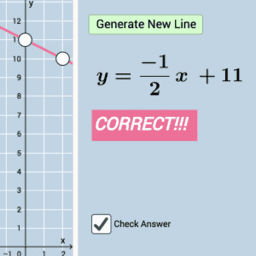### Graphing Linear Equations: Formative Assessment Items

Book

Tim Brzezinski

•### Quiz: Graphing Linear Equations (V3)

Activity

Tim Brzezinski

•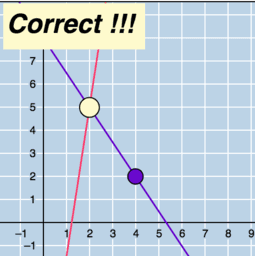### Solving Linear Systems by Graphing: Quiz (V1)

Activity

Tim Brzezinski

•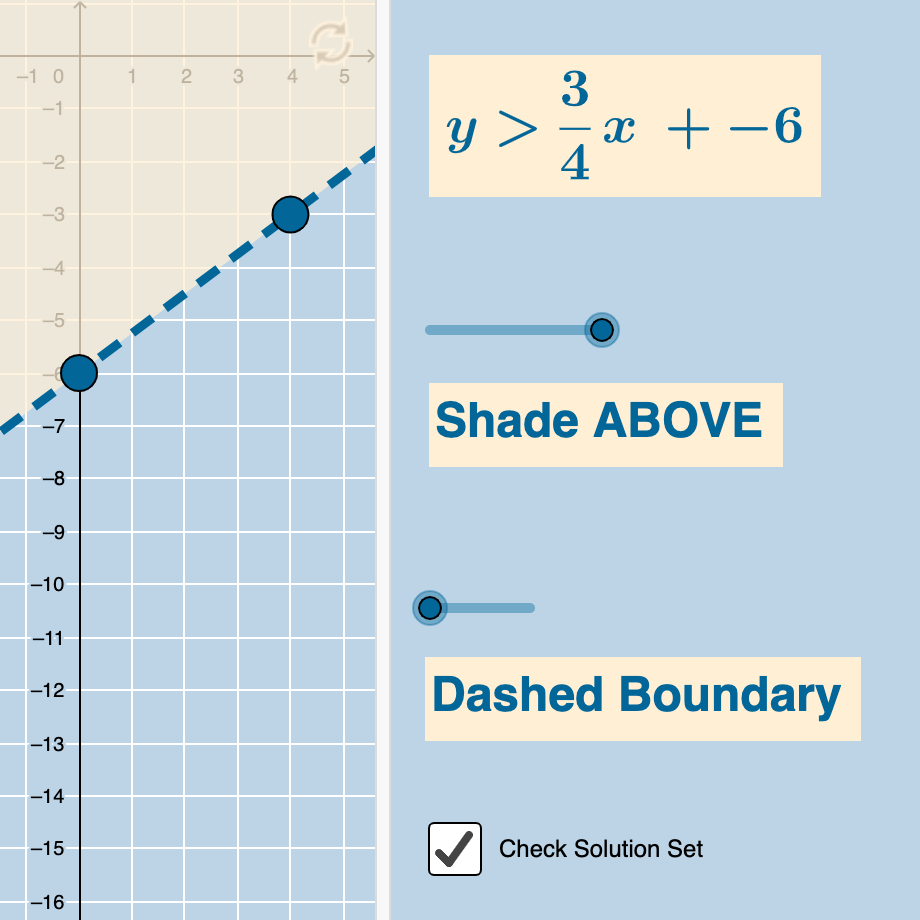### Graphing Linear Inequalities with 2 Variables (Quiz)

Activity

Tim Brzezinski

•### Slope (Quiz) I

Activity

Tim Brzezinski

•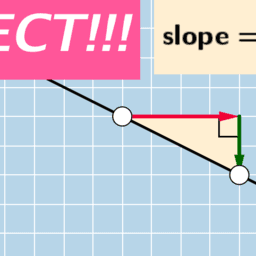### Slope (Quiz) II

Activity

Tim Brzezinski

•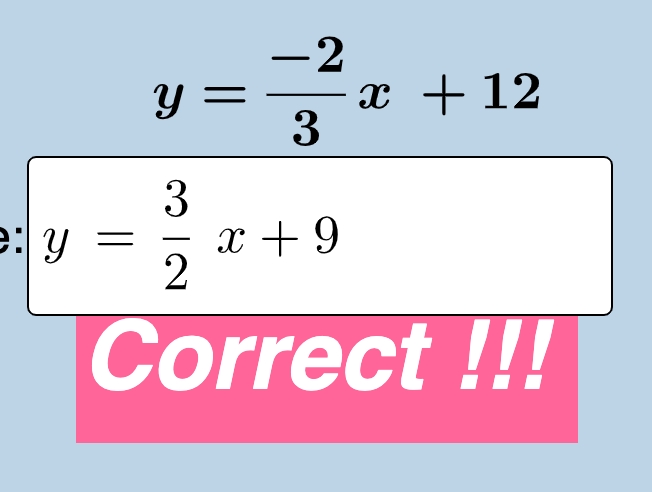### Parallel & Perpendicular (Writing Equations of Lines)

Activity

Tim Brzezinski

•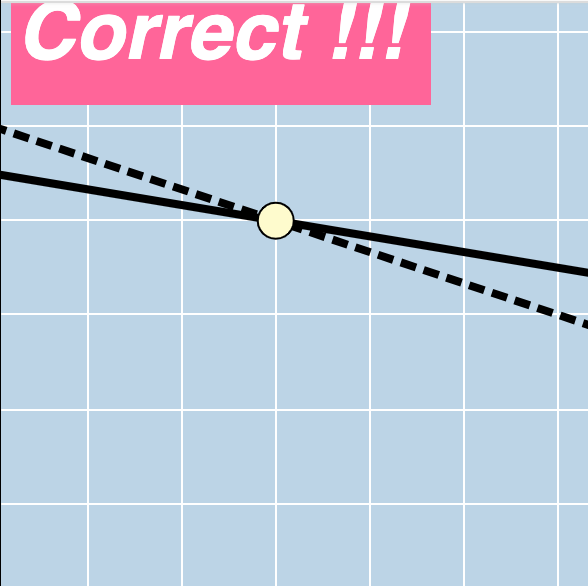### Solving Linear Systems by Graphing: Quiz (V2)

Activity

Tim Brzezinski

•### Writing the Equation of a Line (I-VA)

Activity

Tim Brzezinski

•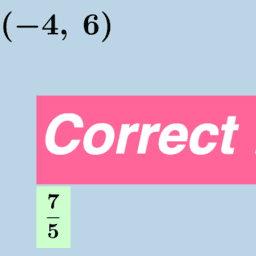### Slope (Quiz) III

Activity

Tim Brzezinski

•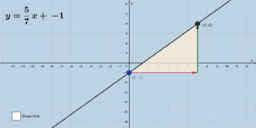### Blueprint Equation of a Line (I)

Activity

Tim Brzezinski

•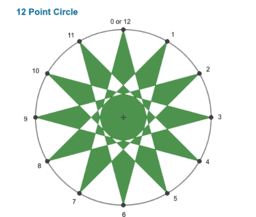### Apps for Formative Assessment Grade 10

Book

Lee W Fisher

•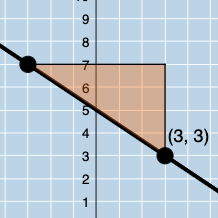### Writing the Equation of a Line Given a Slope & Point (V1)

Activity

Tim Brzezinski

•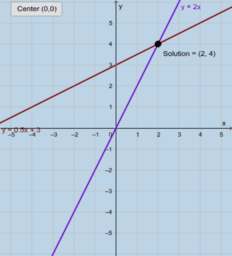### Solving Linear Systems Graphically

Activity

Tim Brzezinski

•### Slope Triangle Rotation (VA)

Activity

Tim Brzezinski

•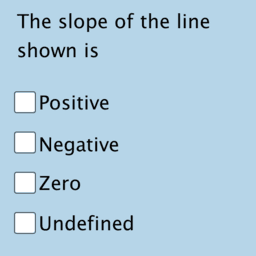### Slope: Quick Intuitive Quiz Questions

Activity

Tim Brzezinski

•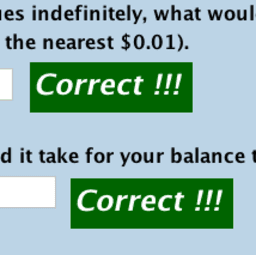### Quiz: Modeling with Linear Functions

Activity

Tim Brzezinski

•### Domain & Range: Illustrated

Activity

Tim Brzezinski

•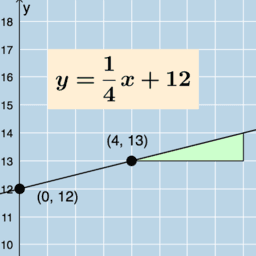### Relating SLOPE and Y-INTERCEPT to the REAL WORLD

Activity

Tim Brzezinski

•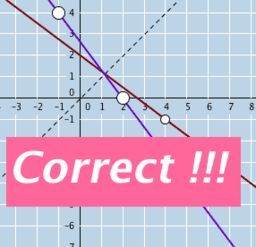### Graphing Inverse Functions: Quiz (1)

Activity

Tim Brzezinski

•### Parallel Lines in the Coordinate Plane (VB)

Activity

Tim Brzezinski

•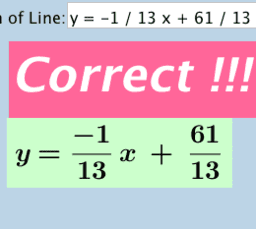### Writing the Equation of a Line Given 2 Points (V2)

Activity

Tim Brzezinski